# The Biaugmented Triangular Prism

The biaugmented triangular prism is the 50th Johnson solid (J50). It has 8 vertices, 17 edges, and 11 faces (10 equilateral triangles and 1 square).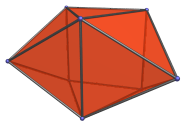The biaugmented triangular prism can be constructed by attaching two square pyramids to two of the square faces of a triangular prism. Or, equivalently, attaching a second square pyramid to the augmented triangular prism (J49). If a third square pyramid is added, the result is the triaugmented triangular prism (J51).

## Projections

Here are some views of the biaugmented triangular prism from various angles:

Projection Description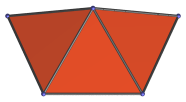Top view.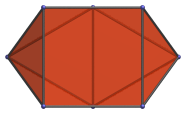Front view.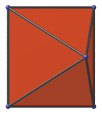Side view perpendicular to axis of symmetry.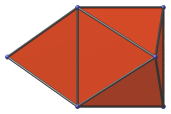Side view, one square face projected to middle vertical edge.

## Coordinates

The Cartesian coordinates of the biaugmented triangular prism with edge length 2 are:

• (±1, −1/√3, ±1)
• (0, 2/√3, ±1)
• (±(1+√6)/2, (1+√6)/(2√3), 0)

Last updated 08 Jun 2018.